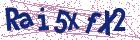# A numerical solution for an inverse heat conduction problem

Document Type : Research Article

Authors

1 Department of Mathematics, Iran University of Science and Technology Narmak, Tehran-16, Iran

2 Faculty of Science, K.N. Toosi University of Technology, Tehran, Iran

Abstract

In this paper, we demonstrate the existence and uniqueness a semianalytical solution of an inverse heat conduction problem (IHCP) in the form: ut = uxx in the domain D = {(x, t)| 0 < x < 1, 0 < t T}, u(x, T) = f(x), u(0, t) = g(t), and ux(0, t) = p(t), for any 0 t T. Some numerical experiments are given in the final section.

Keywords20.1001.1.24236977.2008.1.1.4.9

#### References

 Atkinson Kendal, E., An Introduction to Numerical Analysis, New York, 1964.
 Beck J.V. and Well, B.B., Inverse heat Conduction, Ill-posed Problems, Wi-ley Interscience, New York, 1985.
 Burggraf, O.R., An exact solution of the inverse problem in heat conduction theory and application, J. of Heat Transfer 86(1964), 373-382.
 Cannon, J.R., The One-Dimensinal Heat Equation, Addison-Wesley New York, 1984.